# OS-V: 1300 Flutter Analysis of an AGARD 445.6 Wing

Flutter analysis of an AGARD 445.6 wing model is performed using the PK method.

The results are validated against experimental data from a NASA technical memorandum1.

## Model Files

Before you begin, copy the file(s) used in this problem to your working directory.

## Benchmark Model

Dimension details of the model:
Dimension
Value (m)
Span
0.762
Root Chord Length
0.5587
Tip Chord Length
0.3682
The structural domain consists of a stick model with CQUAD4 elements with linear orthotropic material properties.
E1
3.151E+09
E2
4.162E+08
NU12
0.31
G12
4.392E+08
RHO
381.980
Flutter analysis is performed for set of Mach numbers (M) = {0.35, 0.5, 0.7, 0.9} for a velocity range of [120, 330] m/s. In Figure 15 on page 65, the variation of mass ratios across Mach number suggests difference in flow conditions across the experiments1. Hence, the density ratios were varied for each Mach number in separate simulations.

## Comparison of Normal Modes

Mode shape and mode frequency comparisons are as follows. The results from OptiStruct are in agreement with the reference results1.
Reference results1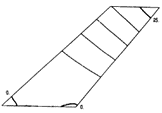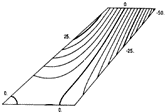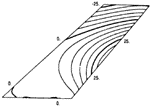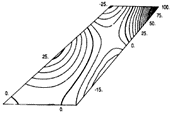Mode 1 F = 9.5992 Hx Mode 2 F = 38.1650 Hz Mode 3 F = 48.3482 Hz Mode 4 F = 91.5448 Hz
Results from OptiStruct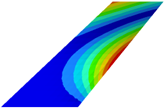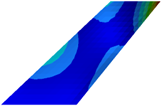Mode 1 F = 9.4589 Hz Mode 2 F = 39.5289 Hz Mode 3 F = 49.2213 Hz Mode 4 F = 94.8019 Hz

## Flutter Analysis

From the .flt file of the first Mach number (M = 0.35) simulation, the flutter point (where damping changes sign) corresponding to the lowest mode is identified as the 2nd mode with a velocity between 128.89 m/s to 131.12 m/s.
Note: By definition, instability (flutter or divergence) occurs when the damping values are zero. At this point, if the frequency is zero, then the instability is due to divergence. Otherwise, the instability is due to flutter.
Plotting the v-g curve, the velocity at this flutter point is 129.417 m/s. This is the most critical flutter point that needs to be avoided for M = 0.35. Plotting the v-f plot for the 1st mode (corresponding to the critical flutter point), the frequency value for 1st mode at a velocity of 129.417 m/s is determined as 24.016 Hz. In the same way, the flutter speed and flutter frequency determination was repeated for M = {0.5, 0.7, 0.9}.

## Comparison of Flutter Speed Coefficient

The flutter speed coefficient $\left(\frac{v}{{b}_{s}{\omega }_{\alpha }\sqrt{\overline{\mu }}}\right)$ is calculated from OptiStruct and plotted against M and compared against the reference plot from Figure 16(a) on page 661.

Where,
$v$
Flutter velocity
${b}_{s}$
Streamwise semi chord length at wing root = $\frac{0.5587}{2}$ m
${\omega }_{\alpha }$
Natural circular frequency of the first uncoupled torsional mode $=2\pi f=2\pi \left(39.5289\right)$ rad/s (This is the 2nd normal mode for this wing)
$\overline{\mu }$
Mass ratio
This value was determined for each Mach number from Figure 15 on page 651
(1)
$\frac{v}{{b}_{s}{\omega }_{\alpha }\sqrt{\overline{\mu }}}$

## Comparison of Flutter Frequency Ratio

The flutter frequency ratio $\left(\frac{\omega }{{\omega }_{\alpha }}\right)$ is calculated from OptiStruct and plotted against M. This is compared against the reference plot from Figure 16(b) on page 671.

(2)
$\frac{\omega }{{\omega }_{\alpha }}$

## Observations

• The flutter speed coefficient and flutter frequency ratio from OptiStruct are in close agreement with the experimental reference data.
• The current support of OptiStruct Aeroelastic Analysis is limited to Subsonic flow (M < 1.0) and hence the simulations were not performed beyond M = 0.9. The support for supersonic regime is planned for a future release and Figure 5 and Figure 6 will be updated with the pertinent data points in this regime.
• In realistic conditions, for M ~ 0.75 and above, local pockets of supersonic flow could occur around the structure. This intermediate regime is denoted as transonic.
• In the flutter speed coefficient versus M plot, the experimental reference data shows a reduction in flutter speed coefficient around M = 1.0 and this is called the transonic dip.
• OptiStruct flutter analysis is capable of capturing the descent of this dip.

## Reference

1 E. Carson Yates Jr, “AGARD Standard Aeroelastic Configurations for Dynamic Response. Candidate Configuration I.-WING 445.6,” NASA Technical Memorandum I00492. 1987